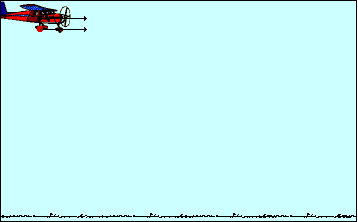## The Plane and The Package

Consider a plane moving with a constant speed at an elevated height above the Earth's surface. In the course of its flight, the plane drops a package from its luggage compartment. What will be the path of the package and where will it be with respect to the plane? And how can the motion of the package be described? The animation below depicts such a situation. The path of the plane and the package are shown; additionally, the velocity components (horizontal and vertical) are represented by arrows in the animation.As can be seen from the above animation, the package follows a parabolic path and remains directly below the plane at all times. As the package falls, it undergoes a vertical acceleration; that is, there is a change in its vertical velocity. This vertical acceleration is attributed to the downward force of gravity which acts upon the package. If the package's motion could be approximated as projectile motion (that is, if the influence of air resistance could be assumed negligible), then there would be no horizontal acceleration. In the absence of horizontal forces, there would be a constant velocity in the horizontal direction. This explains why the package would be located directly under the plane from which it is dropped.

Many would insist that there is a horizontal force acting upon the package since it has a horizontal motion. This is simply not the case. The horizontal motion of the package is the result of its own inertia. When dropped from the plane, the package already possessed a horizontal motion. The package will maintain this state of horizontal motion unless acted upon by a horizontal force. An object in motion will continue in motion with the same speed and in the same direction ... (Newton's first law). Remind yourself continuously: forces do not cause motion; rather, forces cause accelerations.

For more information on physical descriptions of motion, visit The Physics Classroom Tutorial. Detailed information is available there on the following topics:

Acceleration of Gravity

Acceleration of Gravity and the Independence of Mass

Projectiles

Characteristics of a Projectile's Trajectory

Newton's First Law of Motion

Inertia and the State of Motion